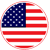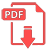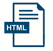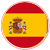# Home | Mathematics | Pre-Algebra

## Trinomial

Before we can get to defining a perfect square trinomial, we need to review some vocabulary.

Perfect squares are numbers or expressions that are the product of a number or expression multiplied to itself. 7 times 7 is 49, so 49 is a perfect square. x squared times x squared equals x to the fourth, so x to the fourth is a perfect square.

Binomials are algrebraic expressions containing only two terms. Example: x + 3
Trinomials are algebraic expressions that contain three terms. Example: 3x2 + 5x - 6

Perfect square trinomials are algebraic expressions with three terms that are created by multiplying a binomial to itself. Example: (3x + 2y)2= 9x2 + 12xy + 4y2

Recognizing when you have these perfect square trinomials will make factoring them much simpler. They are also very helpful when solving and graphing certain kinds of equations.EnglishSpanish